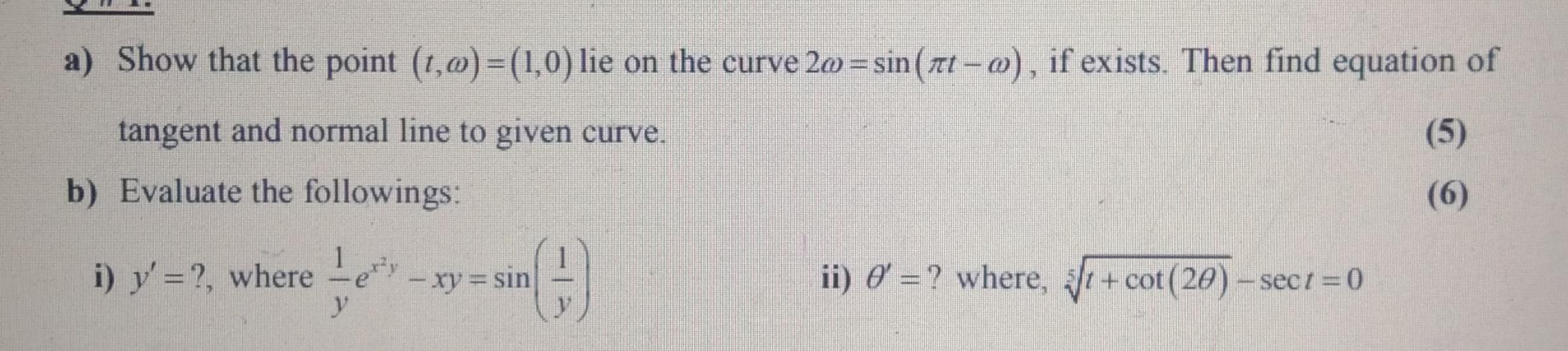### Create an Account

Home / Questions / a) Show that the point (1,0)=(1,0) lie on the curve 20 =sin(att - o), if exists. Then find...

# a) Show that the point (1,0)=(1,0) lie on the curve 20 =sin(att - o), if exists. Then find equation of tangent and normal line to given curve. b) Evaluate the followings: (5) (6) i) y'=?, where e 1 –

a) Show that the point (1,0)=(1,0) lie on the curve 20 =sin(att - o), if exists. Then find equation of tangent and normal line to given curve. b) Evaluate the followings: (5) (6) i) y'=?, where e 1 – xy = = sin sin ii) 6 = ? where, 8t + cot(20) – sect = 0May 17 2021 View more View LessSubscribe To Get Solution